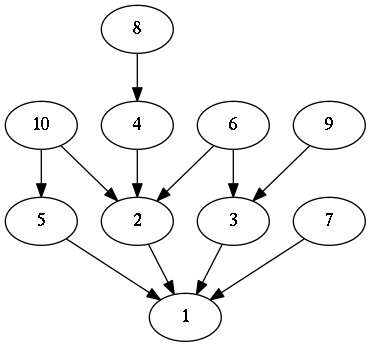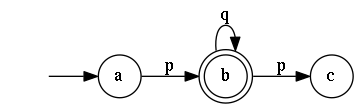# "Haphviz: Graphviz code generation with Haskell"

Date 2015-12-20

I needed some graph visualizations for the chapter on logic and computability in The notes and I really didn't want to write about 20 pieces of repetitively constructed Graphviz code. Less than one week later, Haphviz allowed me to generate those graphs with only a few lines of Haskell code.

Disclaimer: This post assumes that you know how Graphviz' Dot language works. It is written at the time of Haphviz 0.1.4.

### Demonstration

#### Divisors

Ever since I've learned about lattices, I've wanted to generate a Hasse diagram of natural numbers with 'divides' as the partial order. Of course it's impossible to generate an infinite diagram but I would settle for a parametric arbitrarily big diagram.

We'll generate a node in the graph for every natural number starting from $1$. Now, as far as the maths go, there should be an edge between two nodes labeled $a$ and $b$ if $a$ is divisible by $b$ and there doesn't exist a node labeled $c$ that has edges from $a$ and to $b$.

As the math is not the focus of this post, let's see how you would generate code to visualize this graph with Haphviz.

renderToStdOut $graph directed "divisors"$ do
nodes <- forM [1..n] $node . T.pack . show let ns = zip [1..] nodes sequence_ [ni --> nj | (i, ni) <- ns, (j, nj) <- ns, i > j, primary i j] Here the primary :: Integer -> Integer -> Bool function determines wether there should be an edge between two nodes. That wasn't so hard, was it? Running dot on the generate graphiz code with n = 10 yields the following image.#### Finite state automata. Having a Turing-complete encoding system for graph visualization means that we can simplify the process of visualizing graphs with a predictable structure. Haphviz has a module Text.Dot.FSA with a single function fsaGraph that allows you to easily generate graphs for finite state automata. The following code generates Graphviz code for a simple finite state automaton. renderToStdOut$ fsaGraph
[a, b, c]                         -- States
a                                 -- Starting state
[b]                               -- Accepting states
[(a, b, p), (b, b, q), (b, c, p)] -- Edges
where (a, b, c, p, q) = ("a", "b", "c", "p" ,"q")### Design

The design of the internals of this library is based on the design of HaTeX internals. A single data type Dot represents the contents of a graph. Generating graphs by generating a Dot structure is certainly possible but it is a tad tedious.

The DotGen monad allows you to generate graphs in a simpler way. Now you only have to declare how you want to the graph to look and let the combinators take care of constructing a Dot graph.

### Further work

Further extensions of this libraries are certainly possible. Here are some of such extensions I propose. Feel free to have a go at implementing them if you need them.

#### More predictable graph generation

As far as predictable graph generation goes, there is, at the time of writing, only support for finite state automata visualization in Haphviz For The notes, I made a similar piece of code that allowed me to generate Store-Heap model visualizations.

In future work on this library, code could be written to make visualizations of other predictable graphs easier:

• Visualizations of trees from Data.Tree

• Other automata from the domain of computability: Pushdown automata and Turing machines

• Flow networks

• ...

#### A Graph type class

Data.Tree is just one example of a data type that could be represented as a tree. Others may well be visualizable too.

I propose a type class Graph a with a single function graph :: a -> DotGen () that generates its visualization.

#### A DotGen Monad transformer

DotGen is just a monad. It's currently not possible to do IO while generating a graph.

Writing a DotGenT m monad transformer would allow you to compose the graph generation monad with other monads like IO. This would be fairly trivial as DotGen is already a type synonym for StateT State (WriterT Dot Identity).

How I optimized for my exam

Know a technical team that could use strong technical leadership?